# JK Flip Flop Circuit using RS flip flop

## Introduction

In the previous article we discussed about the realization of D flip-flop using SR flip-flop. In this article let’s discuss about the conversion of RS flip-flop into JK flip-flops. We know that Jk flip-flops are one of the important and most widely used flip-flops. So often there is a need for conversion of other type of flip-flops into JK flip-flops. Let us discuss about this conervsion in detail.

## The Transformation

Before dealing with the conversion let’s summarize the truth table of JK flip flop circuits.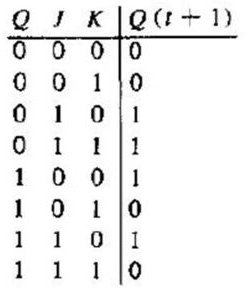From the truth table it’s quite evident that 4 main transitions occur between the current state and next state of JK flip-flop.

They are 0->0; 0->1; 1->0; 1->1.

0-> 0 Transition:

For both the present state and the next state to remain in 0, the J input must remain at 0 and the K input can either be 1 or 0. In simple words, irrespective of the value of K input, the present state and next state remains in 0, if the value of J input is 0.

0->1 Transition:

This is the transition where the present state is 0, but the next state should change to 1. For this transition to occur the value of J input should be 1 and K input can either be 0 or 1. When K value is 0, it is called SET condition (J=1 and K=0, SET condition) and when K value is 1, it is called toggle condition. (J=1 and K=1, TOGGLE condition). So for this transition to occur J has to be high, but K can be either high or low.

1-> 0 Transition:

In this case the present state is 1 and the next state should be 0. For this transition to occur the value of K input should be 1 and the value of J input can be either 0 or 1. Irrespective of the J input, the transition occurs if the value of K remains at 1.

1->1 Transition:

For this transition to occur the value of K should remain low or 0, irrespective of the value of J. J can be either 1 or 0. The value of J will not affect this transition.

From the above transitions we can form the excitation table as shown below.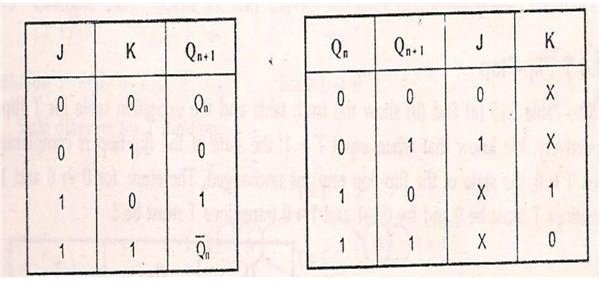The four transitions and excitation table of RS flip-flop is shown below.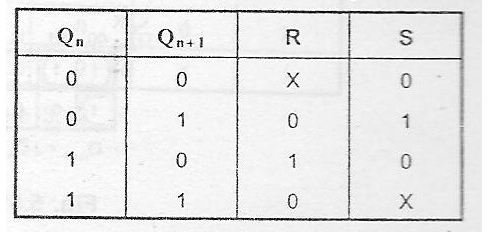## Steps to Convert RS flip-flop to JK Flip-flop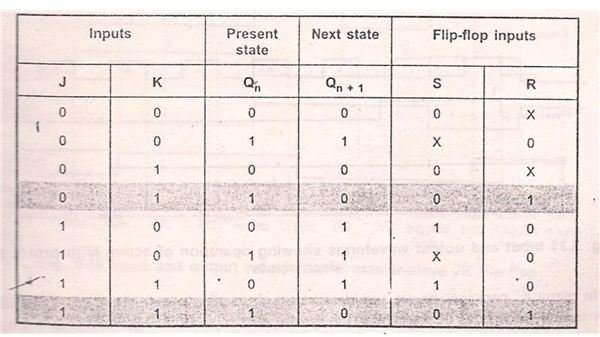• Form the excitation table using S, R, J, K, Q (n) and Q (n+1).
• When J and K inputs are 0 and Q (n) is 0, Then the next state Q (n+1) is also 0. The R an S inputs corresponding to this transition are X and 0 respectively. X is nothing but don’t care condition. In simple words the value of R will not affect the next state of the system. Irrespective of the value of R input, the present state and the next state remains in 0, if the value of S input is 0. Similarly when the inputs J and K are 0 and the present state Q (n) is 1, then the value of the present state is retained for next state too. This is nothing but the 1->1 transition. The S and R input values corresponding to this transition is S=X and R=0. That is S input can be either 0 or 1.
• Consider when J input is 0 and K input is 1. During this condition, the present state is 0 and the same is retained for the next state also. So both the present and next state is 0 and there is a 0-> 0 transition. The value of R and S inputs corresponding to this 0->0 transition is S=0 and R=X. The value of R input does not affect the state of the system. So it is denoted as X. Similarly when J=0 and K=1 and the present state Q (n) is 1, then the next state switches to 0. So in this condition a 1->0 transition occurs. Now the value of RS inputs can be derived from RS excitation table. From the excitation table the value of R and S inputs corresponding to this transition is S=0 and R=1.
• Similarly the entire excitation table for conversion of RS to JK flip-flop can be derived. The excitation table is as shown.
• Draw the K-map for R and S inputs separately using J and K Q (n). Where J and K are inputs of JK flip-flop and Q (n) is the present state of the flip-flop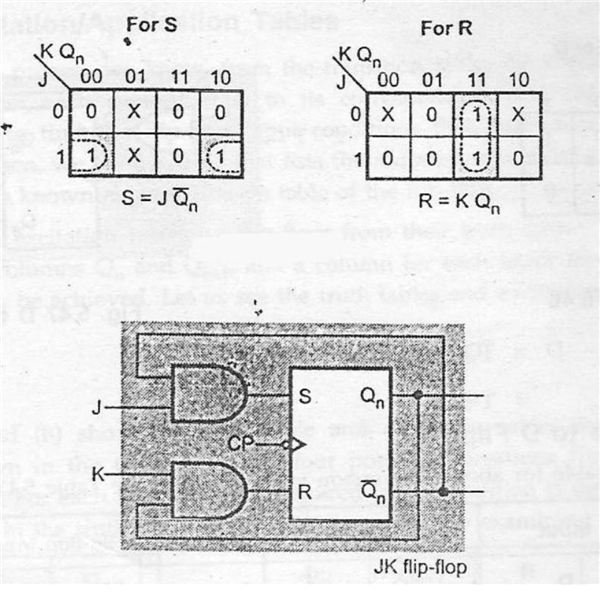• From the K-map we can form a relation between SR and JK flip-flops. A characteristic equation can be obtained which expresses R and S in-terms of J and K. Using this characteristic equation, a logic diagram can be formed which is nothing but the pictorial representation of SR to JK conversion.
• From the K-map the characteristic equation is S=J. Q’ (n) and R=K. Q (n). The Characteristic equation is pictorially represented as logic diagram.

In the next article let us discuss about the other types of realizations.